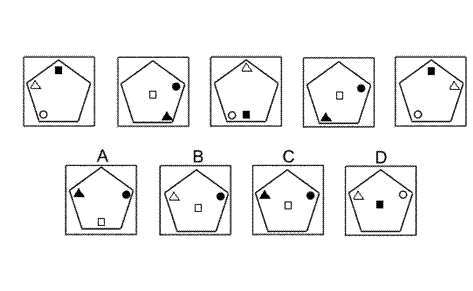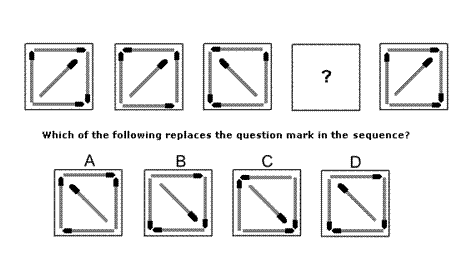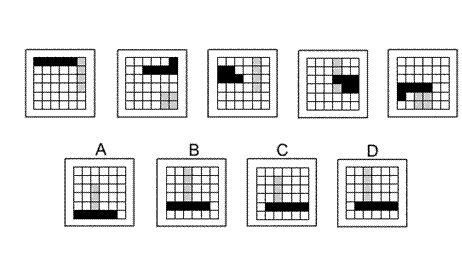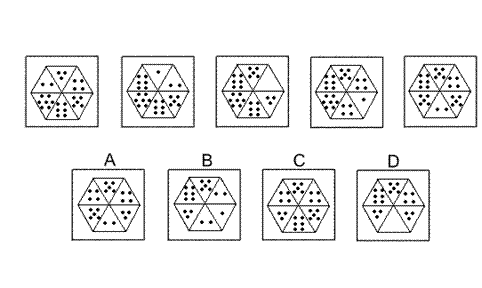Aptitude Tests 4 Me

Non Verbal Logic

Detailed solution

155.Solution: C Explanation: In this question there are three shapes - a triangle, a square and a circle - that move around the pentagon, each according to its own rule. The triangle rotates around the vertices of the pentagon, rotating by 144° anticlockwise each time. The square occupies three positions on the vertical axis of the pentagon, moving up and down. The circle is reflected each time in the axis of symmetry of the pentagon stretching from the top left side to the bottom right vertex. As well as following these rules, the shapes also change colour from one diagram to the next. When all of these rules are applied simultaneously, the correct answer is C.

156.Solution: B Explanation: In this question there are two rules to follow that are applied alternately. The first rule is that the group of matches is reflected in the diagonal stretching from the bottom left corner to the top right corner. The second rule is that the group of matches is rotated about the centre by 90° anticlockwise. To find the missing diagram of the sequence, the first rule should be applied, giving answer B. When the second rule is applied to answer B, the sequence is continued correctly with the final diagram.

157.Solution: D Explanation: In this question there is a 'train' of five black squares and a 'train' of four grey squares that move around the grid. The black 'train' starts in the top left corner and zigzags its way down the rows of the grid, moving forwards 5 places each time. The grey 'train' starts in the top right corner and zigzags its way across the columns of the grid (from left to right), moving forwards 4 places each time. If the two trains occupy the same square, then only the black is visible. When these rules are applied, the next diagram of the sequence is D.

158.159.Solution: C Explanation: In this question there are two rules to follow that are applied simultaneously. The first rule is that the hexagon is rotated by 60° clockwise each time. The second rule is that, after the rotation, the numbers of dots in the top three triangles are each reduced by 1, and the numbers of dots in the bottom three triangles are each increased by 1. When these two rules are applied simultaneously, the correct answer must be C.

 1 2 3 4 5 6 7 8 9 10 11 12 13 14 15 16 17 18 19 20 21 22 23 24 25 26 27 28 29 30 31 32 33 34 35 36 37 38 39 40 41 42 43 44 45 46 47 48 49 50 51 52 53 54 55 56 57 58 59 60 61 62 63 64 65 66 67 68 69 70 71 72 73 74 75 76 77 78 79 80 81 82 83 84 85 86 87 88Passage ReadingVerbal LogicNon Verbal LogicNumerical LogicData InterpretationReasoningAnalytical AbilityBasic NumeracyAbout UsContactPrivacy PolicyMajor TestsFAQ